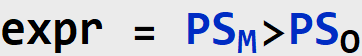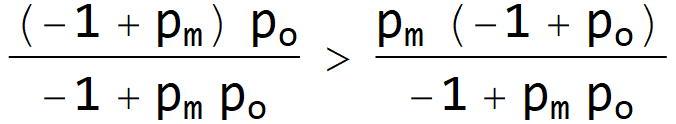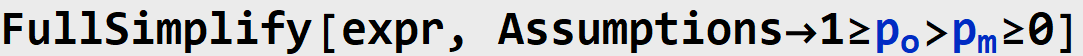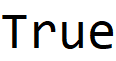Sufficient Causes

A walkthrough of Pearl. J Causal Infer 2019; 20190026.

This paper considers the example of Oxygen, Matches, and Fire in The Book of Why:

“A fire broke out after someone struck a match, and the question is ‘What caused the fire, striking the match or the presence of oxygen in the room?’ Note that both factors are equally necessary, since the fire would not have occurred absent one of them. So, from a purely logical point of view, the two factors are equally responsible for the fire. Why, then, do we consider lighting the match a more reasonable explanation of the fire than the presence of oxygen”

Pearl then introduces the idea of sufficiency: that striking a match is much more likely to be a sufficient condition for the fire than the presence of oxygen.

Let F denote the presence (F=1) or absence (F=1) of a fire, and let M denote whether a match was struck (M=1) or not (M=0). The probability that the match was a sufficient condition can be represented as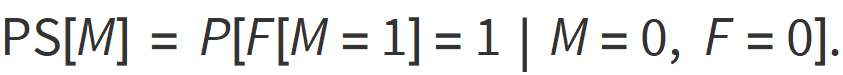Here, F[M=1]=1 denotes a counterfactual. That is, the event that F=1 had only M=1. Note, however, that this probability is conditioned on observing a situation where the match was not struck. Thus, Eq. 1 quantifies the probability that a fire would occur had a match instead been struck.

Now let O be the condition that oxygen is present (O=1) or not (O=0).

Pearl constructs the following graphical model for the situation: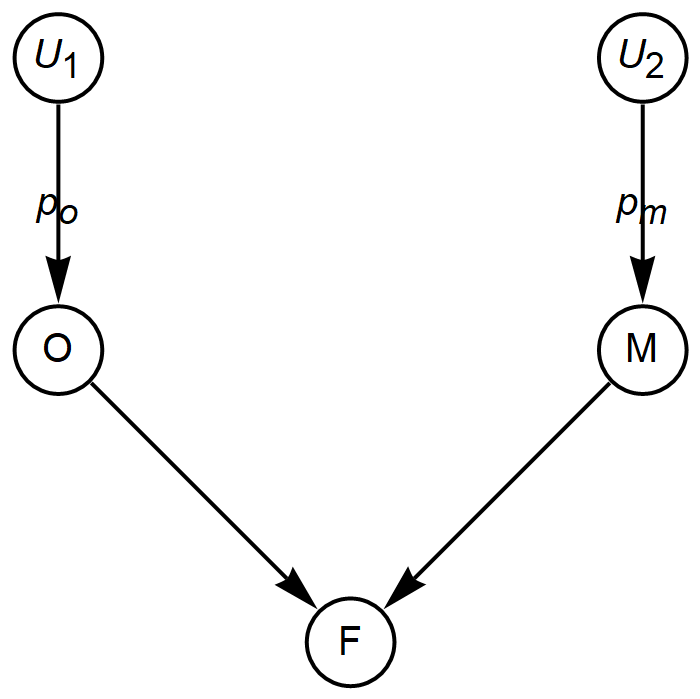Above, theand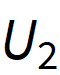nodes are noise that generate random variables M and O with probabilities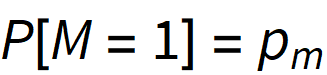and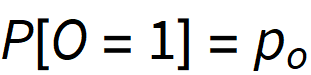, respectively, where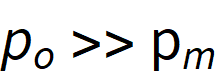. These distributions are Bernoulli: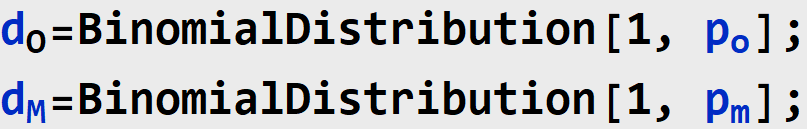We have that F=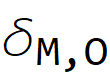, where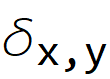is Kronecker’s delta. The joint distribution over M O is as follows: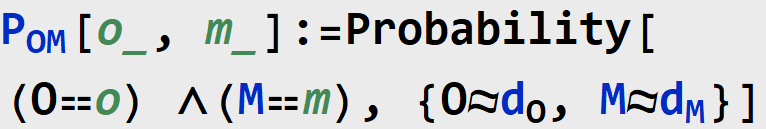The consequent probability distribution over F is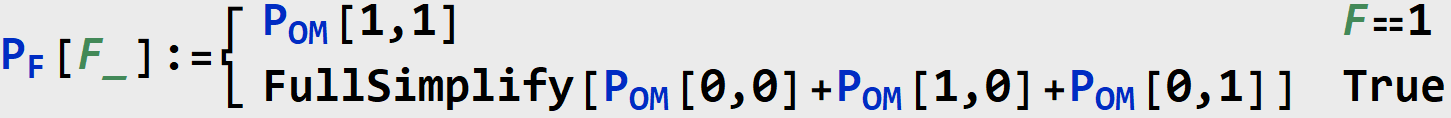Computing the Probability of Sufficiency

Pearl describes a three step procedure for making this counterfactual claim.

1. Abduction: “Updating history in light of available evidence”
2. Action: “Bending the course of history (minimally) to comply with the antecedent”
3. Prediction: “Predicting the outcome based on the updated past and modified model”.

He sets out to compute the following quantities: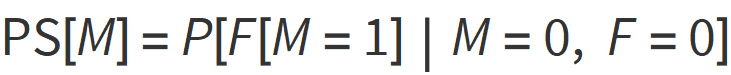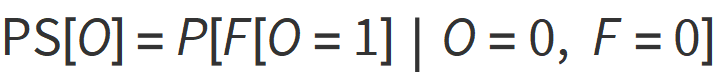Step 1. Abduction

Note that in both cases of Eqs. 2 and 3, we have that F=0. We need to therefore adjust the probabilities of M and O to account only for the situation in which we did not observe a fire.

Pearl does this using Bayes’ rule.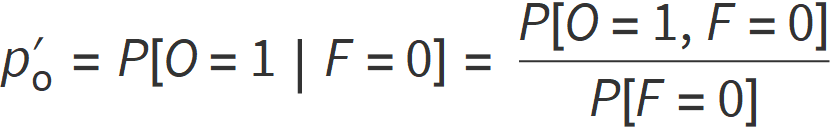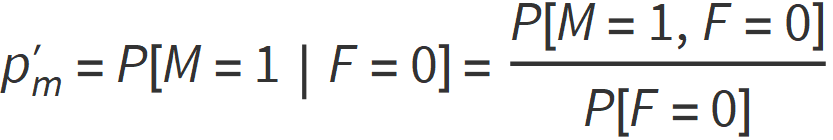Note that P[O=1, F=0] is equivalent to P[O=1, M=0].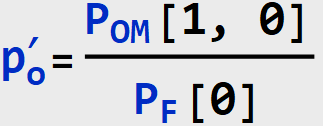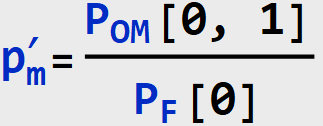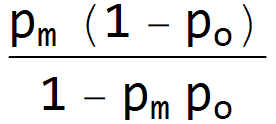We can now update the graphical model with these new probabilities: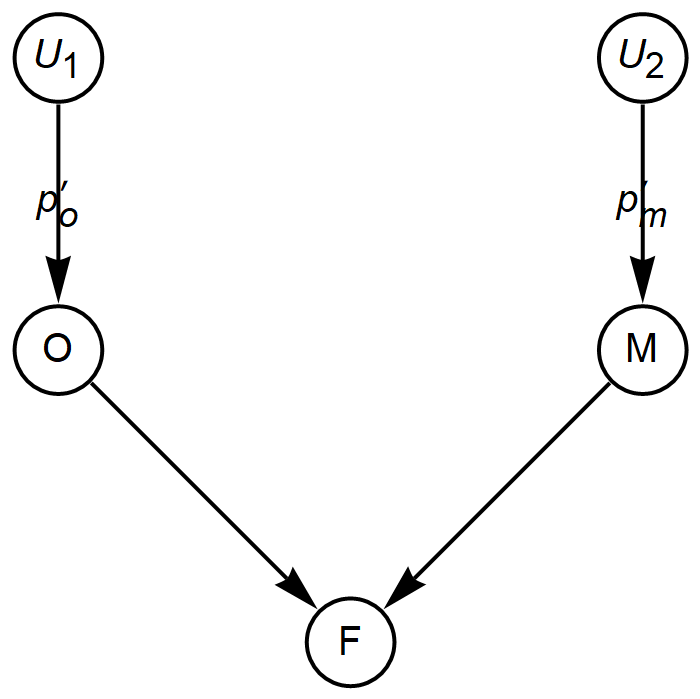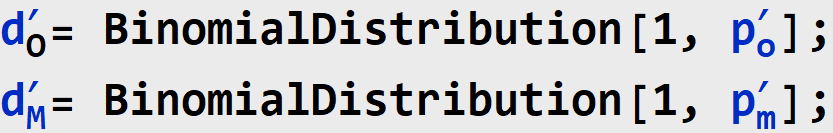The updated joint distribution is therefore as follows: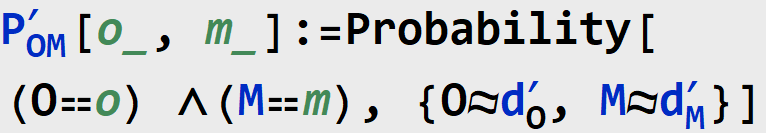Step 2: Action

In this stage, we compute PS[M] and PS[O] by taking the new priors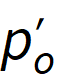and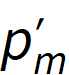, and “simulate the action do[M=1]” (in Pearl’s words from the paper). Graphically, this corresponds to the following models.

Model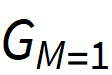is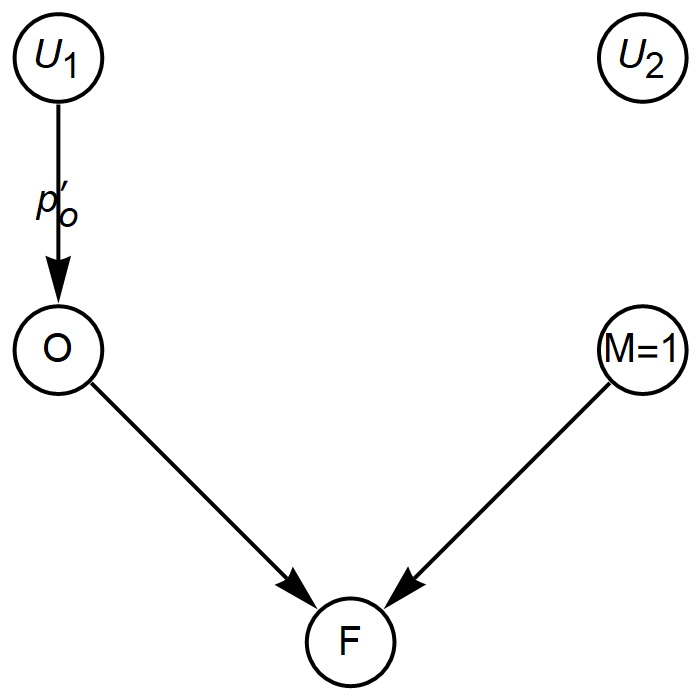and model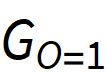is as follows: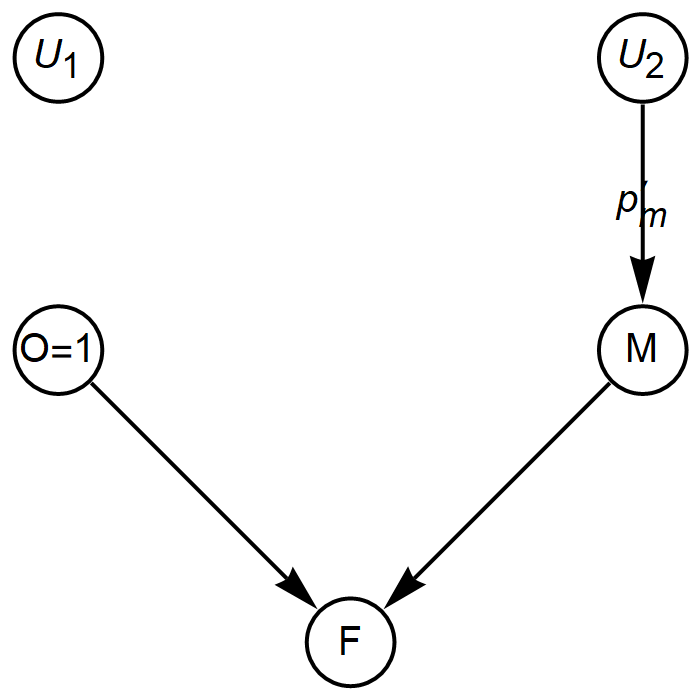Step 3: Prediction

Now, we have to simply compute P[F=1] underand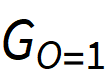, respectively.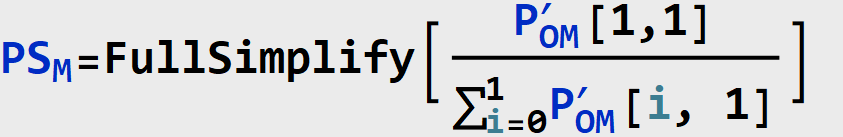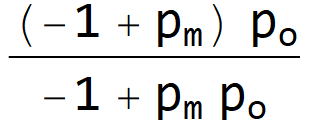Note that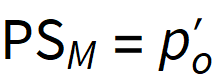: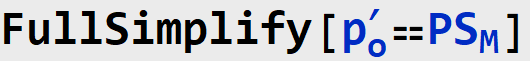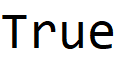Note that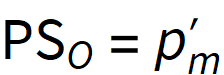: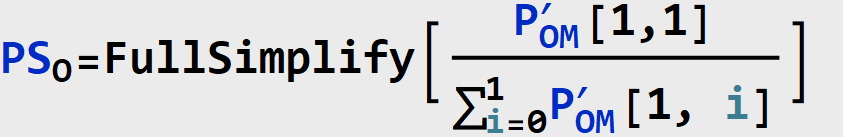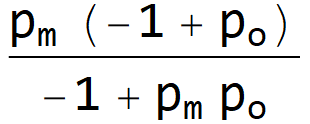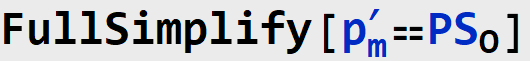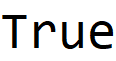So, is the Match a Better Explanation for the Fire?

We can now answer the question of whether a match being lit is a better explanation for the fire than is the presence of oxygen. Namely, the match is a better explanation if its probability of sufficiency PS[M] is greater than that of the oxygen PS[O].